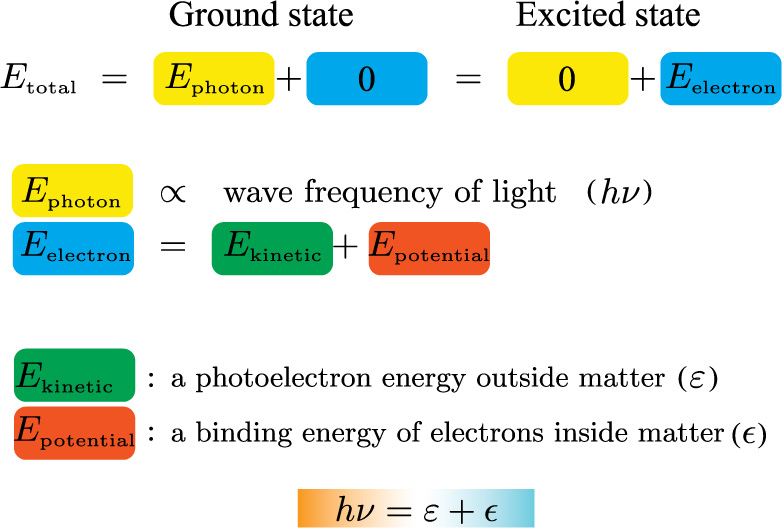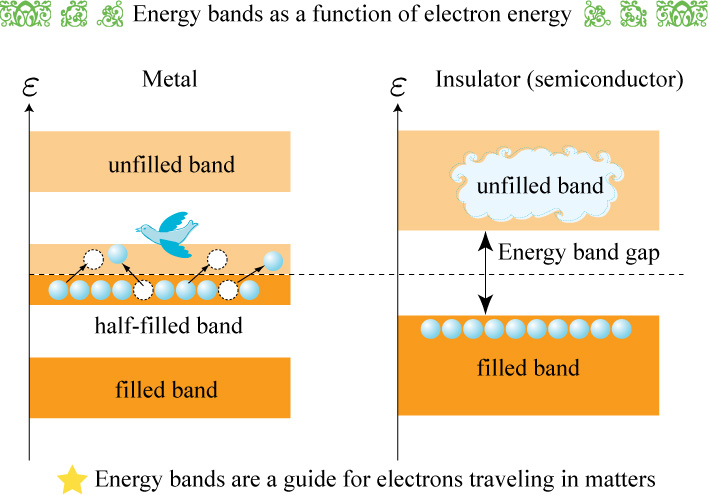### Basic principle of photoemission spectroscopy

[in Thai]

Photoemission is a response of matters by electron emission to the electromagnetic wave. The response of matters is simply due to an energy of the electromagnetic wave transferring to an energy of electrons emitted and/or not. The energy of emitted electrons, photoelectrons, depends on the materials and interactions in it, and so serves as a probe of the materials properties and response. Photoemission spectroscopy (PES) measures the photoelectron energy out of matters.Figure 1: A schematic diagram of photoemission spectroscopy. An incident photon with a quantized energy defined by frequency excites an electron with a binding energy in matter to vacuum. The emitted electron energy analyzed in the hemispherical electric field is a key to understanding the materials.

Even though the energy has various forms such as thermal, kinetic, potential, and so on, the total energy is conserved in the closed system.Figure 2: The law of energy conservation. In a closed system, the energy can change form. Photoemission is a phenomenon that the photon energy changes its form to the electron energy.

The energy of electromagnetic wave was formulated by Einstein on the basis on the experiment performed by Hertz. Einstein quantized the energy as a photon energy, because the energy depends on the frequency of waves. Once, the wave frequency is selected, the energy of electrons is determined.Figure 3: A simple formulation of energies appeared in photoemission spectroscopy.

The electron energy has two components: One is a kinetic energy of photoelectrons, which we can measure. The other is a potential energy leading to a binding energy. The binding energy is a measure of the electron energy in materials, and evaluated from the kinetic energy subtracted from photon energy. The photoelectron numbers scaled in the binding energy, a photoemission spectrum, tells us fruitful information to understanding the materials properties. For instance, a spectrum shows that an energy of electrons in metals is lower than that in insulators.Figure 4:  A photoelectron energy distribution tells us how the material properties appear from viewpoint of physics. One of great achievements by PES explains the electron behavior based on the band structure.

The energy band gap in insulators is a key parameter to the development for next generation electronic devices. The finding for new materials based on this parameter is one of our important targets.

Synchrotron Light Research Institute,